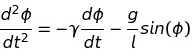# Computation Contest #2 [6 SBI]Here you can solve interesting problems using whatever programming language you like. Also you will earn SBI and sometimes STEM by doing so.
Also you might learn new things by doing so.
The tasks will be rather hard to solve without a programmable computer and some programming skills, but if you want to add a few million numbers by hand or similar, I would still give you the reward.
↓↑↓↑↓↑↓↑↓↑↓↑↓↑↓↑↓↑↓↑↓↑↓↑↓↑↓↑↓↑↓↑↓↑↓↑↓↑↓↑↓↑↓↑↓↑↓↑↓

# Rules

#### In order to get accepted you need to somehow attach your code.

↓↑↓↑↓↑↓↑↓↑↓↑↓↑↓↑↓↑↓↑↓↑↓↑↓↑↓↑↓↑↓↑↓↑↓↑↓↑↓↑↓↑↓↑↓↑↓↑↓

# Problem

Today I want you to write some kind of physics simulation:
You should simulate a pendulum!
A pendulum can be described by the following equation, where phi is the current angle, gamma is some dampening constant and l is the length:To solve this you can approximate dt with some small value and use the formula to calculate the change in velocity and position each time step.

Now you have to solve 2 problems that show that you made a working model:

1. Consider a pendulum of length 1m and dampening constant 0.01/s and starting velocity of 360 °/s starting at a starting angle of 0°.
How many times will it circle around until it settles to a swinging movement.
2. What would the dampening constant need to be for the pendulum to stop after completing only 3 circles?

↓↑↓↑↓↑↓↑↓↑↓↑↓↑↓↑↓↑↓↑↓↑↓↑↓↑↓↑↓↑↓↑↓↑↓↑↓↑↓↑↓↑↓↑↓↑↓↑↓

To everyone who already participated in a past contest, come back today and try a new problem(tell me if you don't want to be tagged):
@crokkon @kaeserotor @tonimontana

In case no one gets a result(which I doubt), I will give away the prize to the person who makes the most constructive description why the problem is too hard in your opinion.

↓↑↓↑↓↑↓↑↓↑↓↑↓↑↓↑↓↑↓↑↓↑↓↑↓↑↓↑↓↑↓↑↓↑↓↑↓↑↓↑↓↑↓↑↓↑↓↑↓

@contrabourdon sponsors my contests with 2 STEEM weekly.
You can support him by using a witness vote on untersatz, so he can further support this and other contests.

ok so here is my solution:

``````import numpy as np

class calc:
def __init__(self):
self.l=1
self.dt=0.001
self.v=360
self.g=9.81
self.phi=0
self.a=1

def acc(self, gamma):
self.a= -gamma*self.v-self.g/self.l*np.sin(self.phi)

def vel(self):
self.v=self.v+self.a*self.dt

def ang(self):
self.phi=self.phi+self.v*self.dt

c=calc()
t=0
acclist=[]
vellist=[]
anglist=[]
time=[]

gamma=0.01

while t<1000:
c.acc(gamma)
c.vel()
c.ang()

acclist.append(c.a)
vellist.append(c.v)
anglist.append(c.phi)
time.append(t)

while t<max_time:
c.acc(gamma)
c.vel()
c.ang()

anglist.append(c.phi)
if round(max(anglist)/360)>3:
steps=[gamma,steps]
else:
steps=[steps,gamma]

print(gamma)

c=calc()
t=0
acclist=[]
vellist=[]
anglist=[]
time=[]

while t<1000:
c.acc(gamma)
c.vel()
c.ang()
t+=0.001
acclist.append(c.a)
vellist.append(c.v)
anglist.append(c.phi)
time.append(t)

print(max(anglist)/360.0)``````

The output gives:
98.79413003199605
450.64399999625556
0.390625
2.536477974411837

1. With gamma=0.01 the pendulum does 98 full circles
2. Using simple iterations, I get a value of around 0.390625 if only 3 circles shall be completed. However, it's strange that the pendulum would stop at just above 2.5 circles, because this is just after the highest point. So maybe my calculations are wrong or at least i am very close to the lower bound
04.11.2019 22:21
1

You should be careful with degrees and radians. Your whole program works with degrees EXCEPT the numpy.sin(x) function which only accepts radians. So I think you just need to replace 360° with 2π everywhere.

05.11.2019 18:47
0

You are right, I adjusted it and also had to manipulate the max_time approach. Now my results are:

1. 0.49420894870809245 (stops juuuust before the tipping point)
2. For gamma =0.00146484375 it does 3.492783208842303 rotations. of course way too many numbers behing the comma, just copy-pasted it here
05.11.2019 19:58
1

In my simulation it makes one round.

It seems I took the worst example I could possibly take :(

The difference between your `g = 9.81 m/s²` and the real average `g = 9.80665 m/s²` decides wether it succeeds over the tipping point or stops right before it.

No need for you to change anything. This rounding error is ignored since g is not that constant all over earth.

05.11.2019 20:51
2

Yes I remembered the value of g from school and already wondered where you are from and if g is different there :D

05.11.2019 20:53
0